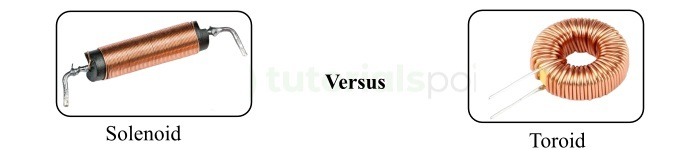# Difference between Solenoid and Toroid

Both solenoid and toroid are coils made of conductor wires and are used in a variety of electrical and electronic circuits to produce a magnetic field with help of electric current.

The fundamental difference between solenoid and toroid is that the solenoid is a cylindrical shaped electromagnetic coil used to generate a magnetic field outside it, while a toroid is a circular (doughnut) shaped electromagnetic coil used to produce a magnetic field within itself.In this article, we will learn the important differences between solenoid and toroid, but before discussing the differences, let us first have a look into their basics individually.

## What is a Solenoid?

An electromagnetic coil made by wounding a conductor wire of finite length around a cylindrical metal core is called a solenoid.

When an electric current is made to flow through the conductor coil, a magnetic field is generated outside the coil. Where, the strength of the magnetic field is directly proportional to the amount of current and the number of turn in the coil.

Solenoids are widely used in a variety of electromechanical systems, like door and window lock, automobile starter motors, valves, relays, static switches, etc.

Solenoid is a simple and efficient device for converting electrical energy into mechanical energy, which makes it an important component of many electromechanical systems like robotics, automation, electric vehicles, etc.

## What is a Toroid?

A circular shaped (doughnut shaped) electromagnetic coil made up of conductor wire to generate a magnetic field is called a toroid. Toroid is a widely used electromagnetic coil in a variety of electronic devices.

A toroid is typical made up of a circular core of ferromagnetic material and insulated conductor wire like copper. The circular or ring shape of the toroid made it more efficient in terms of magnetic field production. When an electric current is made to flow through the coil, a magnetic field is generate which is confined to the toroid magnetic core.

Another major advantage of toroidal construction is low electromagnetic interference with other electronic components. Toroid is widely used in wider range of applications where, compact size, high magnetic efficiency, low electromagnetic interference, more precise control of electrical energy is needed.

After discussing the basics of solenoid and toroid, let us now understand their important differences.

## Difference between Solenoid and Toroid

The important differences between solenoid and toroid are highlighted in the following table:

Parameter

Solenoid

Toroid

Basic

An electromagnetic coil made in a cylindrical shape by wrapping a conductor wire around a magnetic core is referred to as a solenoid.

An electromagnetic coil made in a doughnut shape by wrapping an insulated conductor wire around a ring-shaped core is referred to as a toroid.

Shape

Solenoid has cylindrical shape.

Toroid has a circular or ring or doughnut shape.

Magnetic field

Solenoid produces a magnetic field outside it, and it is concentrated at the center of the coil.

Toroid produces a magnetic field within, which is concentrated within the toroid itself.

Uniformity of magnetic field

Solenoid has a uniform magnetic field inside it.

Toroid does not have a uniform magnetic field inside it.

Inductance

For the same size, a solenoid has a lower inductance.

Toroid has a comparatively higher inductance.

Winding

Solenoid has a winding wound in a linear manner.

Toroid has a winding of insulated wire wound in a circular manner.

Cost

Solenoid is less expensive due to its simple design.

Toroid is relatively costlier due to complex design.

Number of turns

For the same inductance, a solenoid has a greater number of turns.

Toroid has a less number of turns.

Magnetic field formula

The magnetic field of a solenoid is given by,$B = \frac{\mu NI}{I}$

The magnetic field of a toroid is given by,$B = \frac{\mu NI}{2\pi r}$

Efficiency

Solenoid is comparatively less efficient, as it create magnetic field outside, increasing energy losses.

Toroid is more energy efficient, as it creates magnetic field within it.

Electromagnetic interference

Solenoid causes more electromagnetic interference with other electronic devices.

Toroid causes less electromagnetic interference with other electronic devices in the circuit.

Applications

Solenoid is widely used in power switches, electrically operated valves, door locking systems, printers, fuel injectors in automobiles, etc.

Toroid is used in electronic circuits, mobile chargers, medical instruments, telecommunication systems, amplifiers, electronics clutches and brakes, etc.

## Conclusion

Both solenoid and toroid are the type of electromagnetic devices used in a variety of electronic applications. But they are completely different from each other in several aspects as described in the above table. The most significant difference between solenoid and toroid is that solenoid is a cylindrical shaped electromagnetic coil, while a toroid is a ring-shaped electromagnetic coil.

Updated on: 16-May-2023

275 Views